# 6.2 Determining volumes by slicing  (Page 7/12)

 Page 7 / 12

A tetrahedron with a base side of 4 units, as seen here.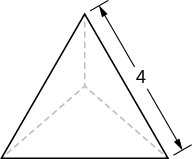$\frac{32}{3\sqrt{2}}$ units 3

A pyramid with height 5 units, and an isosceles triangular base with lengths of 6 units and 8 units, as seen here.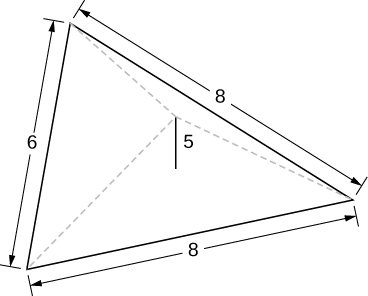A cone of radius $r$ and height $h$ has a smaller cone of radius $r\text{/}2$ and height $h\text{/}2$ removed from the top, as seen here. The resulting solid is called a frustum .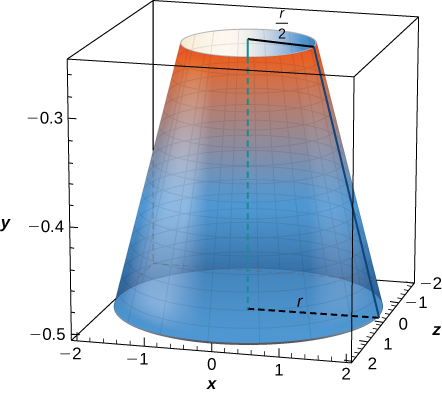$\frac{7\pi }{12}h{r}^{2}$ units 3

For the following exercises, draw an outline of the solid and find the volume using the slicing method.

The base is a circle of radius $a.$ The slices perpendicular to the base are squares.

The base is a triangle with vertices $\left(0,0\right),\left(1,0\right),$ and $\left(0,1\right).$ Slices perpendicular to the xy -plane are semicircles.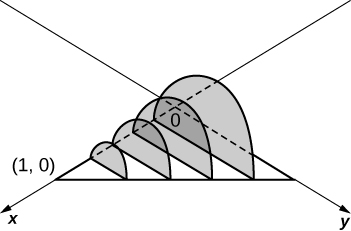$\frac{\pi }{24}$ units 3

The base is the region under the parabola $y=1-{x}^{2}$ in the first quadrant. Slices perpendicular to the xy -plane are squares.

The base is the region under the parabola $y=1-{x}^{2}$ and above the $x\text{-axis}\text{.}$ Slices perpendicular to the $y\text{-axis}$ are squares.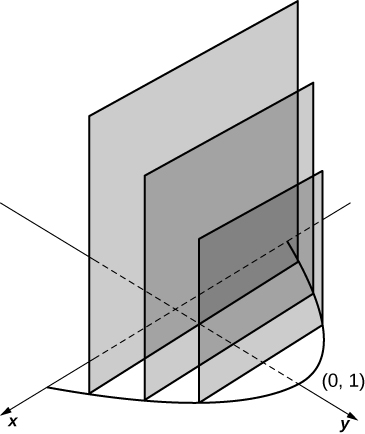$2$ units 3

The base is the region enclosed by $y={x}^{2}$ and $y=9.$ Slices perpendicular to the x -axis are right isosceles triangles.

The base is the area between $y=x$ and $y={x}^{2}.$ Slices perpendicular to the x -axis are semicircles.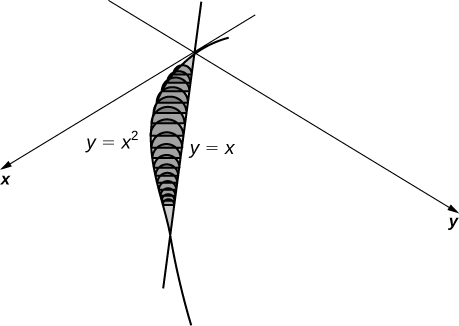$\frac{\pi }{240}$ units 3

For the following exercises, draw the region bounded by the curves. Then, use the disk method to find the volume when the region is rotated around the x -axis.

$x+y=8,x=0,\phantom{\rule{0.2em}{0ex}}\text{and}\phantom{\rule{0.2em}{0ex}}y=0$

$y=2{x}^{2},x=0,x=4,\phantom{\rule{0.2em}{0ex}}\text{and}\phantom{\rule{0.2em}{0ex}}y=0$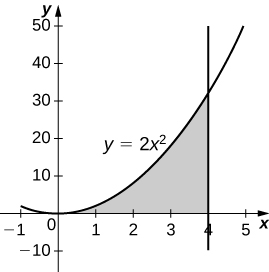$\frac{4096\pi }{5}$ units 3

$y={e}^{x}+1,x=0,x=1,\phantom{\rule{0.2em}{0ex}}\text{and}\phantom{\rule{0.2em}{0ex}}y=0$

$y={x}^{4},x=0,\phantom{\rule{0.2em}{0ex}}\text{and}\phantom{\rule{0.2em}{0ex}}y=1$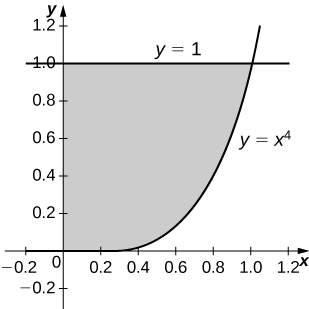$\frac{8\pi }{9}$ units 3

$y=\sqrt{x},x=0,x=4,\phantom{\rule{0.2em}{0ex}}\text{and}\phantom{\rule{0.2em}{0ex}}y=0$

$y=\text{sin}\phantom{\rule{0.2em}{0ex}}x,y=\text{cos}\phantom{\rule{0.2em}{0ex}}x,\phantom{\rule{0.2em}{0ex}}\text{and}\phantom{\rule{0.2em}{0ex}}x=0$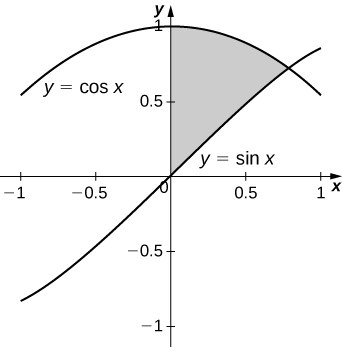$\frac{\pi }{2}$ units 3

$y=\frac{1}{x},x=2,\phantom{\rule{0.2em}{0ex}}\text{and}\phantom{\rule{0.2em}{0ex}}y=3$

${x}^{2}-{y}^{2}=9\phantom{\rule{0.2em}{0ex}}\text{and}\phantom{\rule{0.2em}{0ex}}x+y=9,y=0\phantom{\rule{0.2em}{0ex}}\text{and}\phantom{\rule{0.2em}{0ex}}x=0$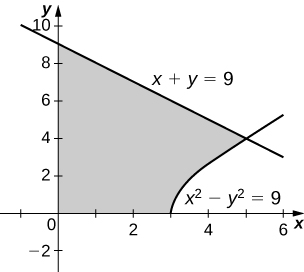$207\pi$ units 3

For the following exercises, draw the region bounded by the curves. Then, find the volume when the region is rotated around the y -axis.

$y=4-\frac{1}{2}x,x=0,\phantom{\rule{0.2em}{0ex}}\text{and}\phantom{\rule{0.2em}{0ex}}y=0$

$y=2{x}^{3},x=0,x=1,\phantom{\rule{0.2em}{0ex}}\text{and}\phantom{\rule{0.2em}{0ex}}y=0$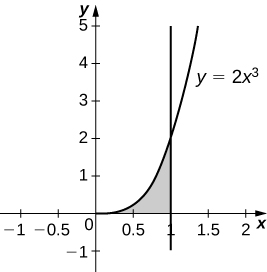$\frac{4\pi }{5}$ units 3

$y=3{x}^{2},x=0,\phantom{\rule{0.2em}{0ex}}\text{and}\phantom{\rule{0.2em}{0ex}}y=3$

$y=\sqrt{4-{x}^{2}},y=0,\phantom{\rule{0.2em}{0ex}}\text{and}\phantom{\rule{0.2em}{0ex}}x=0$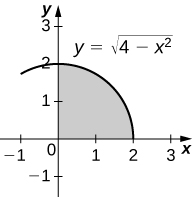$\frac{16\pi }{3}$ units 3

$y=\frac{1}{\sqrt{x+1}},x=0,\phantom{\rule{0.2em}{0ex}}\text{and}\phantom{\rule{0.2em}{0ex}}x=3$

$x=\text{sec}\left(y\right)\phantom{\rule{0.2em}{0ex}}\text{and}\phantom{\rule{0.2em}{0ex}}y=\frac{\pi }{4},y=0\phantom{\rule{0.2em}{0ex}}\text{and}\phantom{\rule{0.2em}{0ex}}x=0$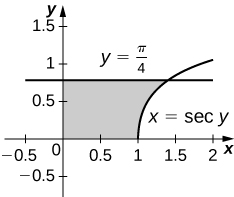$\pi$ units 3

$y=\frac{1}{x+1},x=0,\phantom{\rule{0.2em}{0ex}}\text{and}\phantom{\rule{0.2em}{0ex}}x=2$

$y=4-x,y=x,\phantom{\rule{0.2em}{0ex}}\text{and}\phantom{\rule{0.2em}{0ex}}x=0$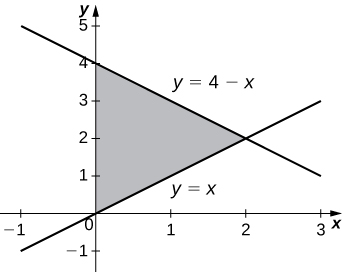$\frac{16\pi }{3}$ units 3

For the following exercises, draw the region bounded by the curves. Then, find the volume when the region is rotated around the x -axis.

$y=x+2,y=x+6,x=0,\phantom{\rule{0.2em}{0ex}}\text{and}\phantom{\rule{0.2em}{0ex}}x=5$

$y={x}^{2}\phantom{\rule{0.2em}{0ex}}\text{and}\phantom{\rule{0.2em}{0ex}}y=x+2$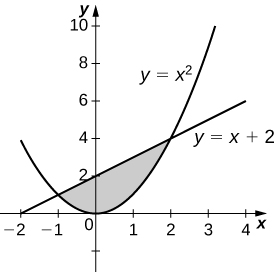$\frac{72\pi }{5}$ units 3

${x}^{2}={y}^{3}\phantom{\rule{0.2em}{0ex}}\text{and}\phantom{\rule{0.2em}{0ex}}{x}^{3}={y}^{2}$

$y=4-{x}^{2}\phantom{\rule{0.2em}{0ex}}\text{and}\phantom{\rule{0.2em}{0ex}}y=2-x$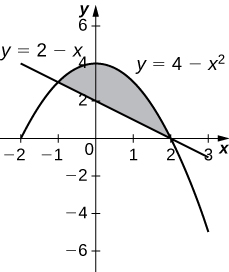$\frac{108\pi }{5}$ units 3

[T] $y=\text{cos}\phantom{\rule{0.2em}{0ex}}x,y={e}^{\text{−}x},x=0,\phantom{\rule{0.2em}{0ex}}\text{and}\phantom{\rule{0.2em}{0ex}}x=1.2927$

$y=\sqrt{x}\phantom{\rule{0.2em}{0ex}}\text{and}\phantom{\rule{0.2em}{0ex}}y={x}^{2}$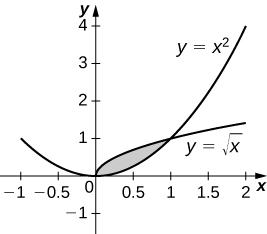$\frac{3\pi }{10}$ units 3

$y=\text{sin}\phantom{\rule{0.2em}{0ex}}x\text{,}\phantom{\rule{0.2em}{0ex}}y=5\phantom{\rule{0.2em}{0ex}}\text{sin}\phantom{\rule{0.2em}{0ex}}x,x=0\phantom{\rule{0.2em}{0ex}}\text{and}\phantom{\rule{0.2em}{0ex}}x=\pi$

$y=\sqrt{1+{x}^{2}}\phantom{\rule{0.2em}{0ex}}\text{and}\phantom{\rule{0.2em}{0ex}}y=\sqrt{4-{x}^{2}}$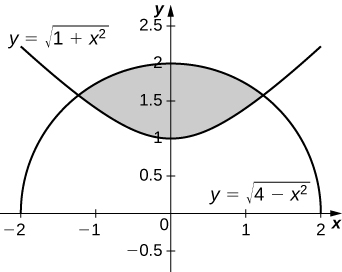$2\sqrt{6}\pi$ units 3

For the following exercises, draw the region bounded by the curves. Then, use the washer method to find the volume when the region is revolved around the y -axis.

$y=\sqrt{x},x=4,\phantom{\rule{0.2em}{0ex}}\text{and}\phantom{\rule{0.2em}{0ex}}y=0$

$y=x+2,y=2x-1,\phantom{\rule{0.2em}{0ex}}\text{and}\phantom{\rule{0.2em}{0ex}}x=0$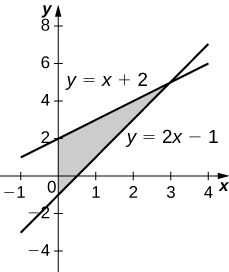$9\pi$ units 3

$y=\sqrt{x}\phantom{\rule{0.2em}{0ex}}\text{and}\phantom{\rule{0.2em}{0ex}}y={x}^{3}$

$x={e}^{2y},x={y}^{2},y=0,\phantom{\rule{0.2em}{0ex}}\text{and}\phantom{\rule{0.2em}{0ex}}y=\text{ln}\left(2\right)$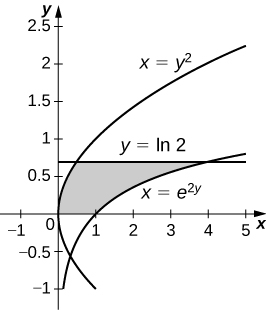$\frac{\pi }{20}\left(75-4\phantom{\rule{0.2em}{0ex}}{\text{ln}}^{5}\left(2\right)\right)$ units 3

$x=\sqrt{9-{y}^{2}},x={e}^{\text{−}y},y=0,\phantom{\rule{0.2em}{0ex}}\text{and}\phantom{\rule{0.2em}{0ex}}y=3$

Yogurt containers can be shaped like frustums. Rotate the line $y=\frac{1}{m}x$ around the y -axis to find the volume between $y=a\phantom{\rule{0.2em}{0ex}}\text{and}\phantom{\rule{0.2em}{0ex}}y=b.$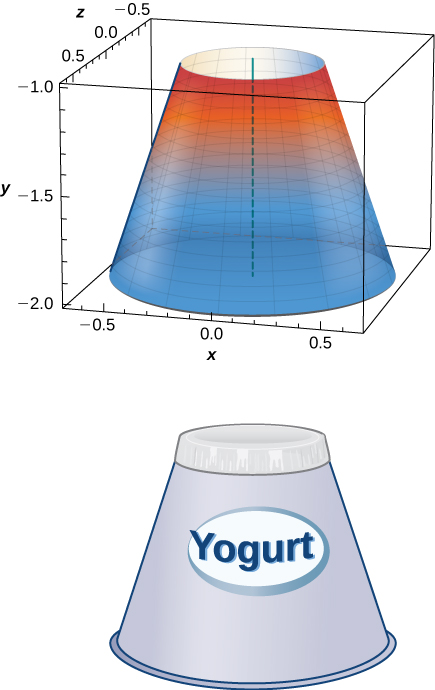$\frac{{m}^{2}\pi }{3}\left({b}^{3}-{a}^{3}\right)$ units 3

Rotate the ellipse $\left({x}^{2}\text{/}{a}^{2}\right)+\left({y}^{2}\text{/}{b}^{2}\right)=1$ around the x -axis to approximate the volume of a football, as seen here.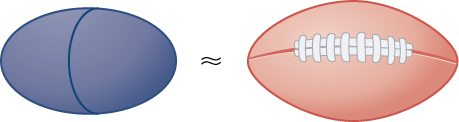Rotate the ellipse $\left({x}^{2}\text{/}{a}^{2}\right)+\left({y}^{2}\text{/}{b}^{2}\right)=1$ around the y -axis to approximate the volume of a football.

$\frac{4{a}^{2}b\pi }{3}$ units 3

A better approximation of the volume of a football is given by the solid that comes from rotating $y=\text{sin}\phantom{\rule{0.2em}{0ex}}x$ around the x -axis from $x=0$ to $x=\pi .$ What is the volume of this football approximation, as seen here?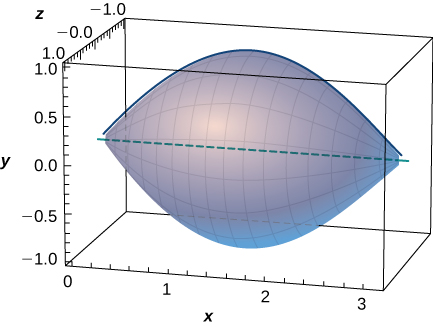What is the volume of the Bundt cake that comes from rotating $y=\text{sin}\phantom{\rule{0.2em}{0ex}}x$ around the y -axis from $x=0$ to $x=\pi ?$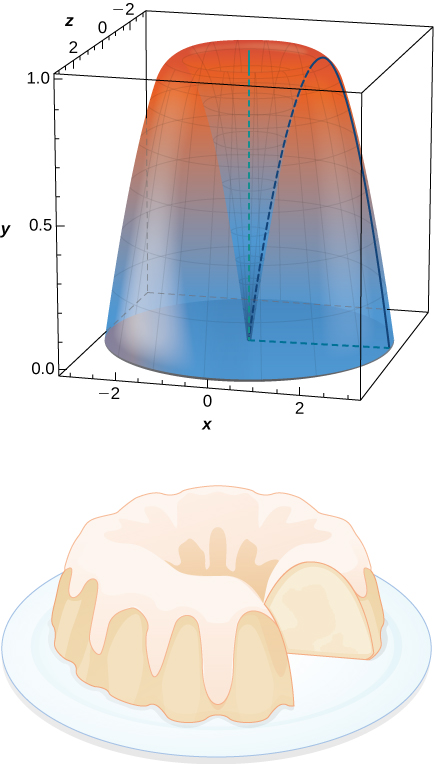$2{\pi }^{2}$ units 3

For the following exercises, find the volume of the solid described.

The base is the region between $y=x$ and $y={x}^{2}.$ Slices perpendicular to the x -axis are semicircles.

The base is the region enclosed by the generic ellipse $\left({x}^{2}\text{/}{a}^{2}\right)+\left({y}^{2}\text{/}{b}^{2}\right)=1.$ Slices perpendicular to the x -axis are semicircles.

$\frac{2a{b}^{2}\pi }{3}$ units 3

Bore a hole of radius $a$ down the axis of a right cone and through the base of radius $b,$ as seen here.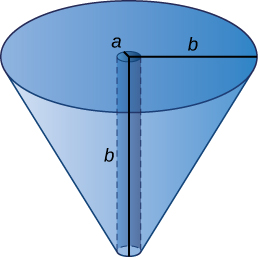Find the volume common to two spheres of radius $r$ with centers that are $2h$ apart, as shown here.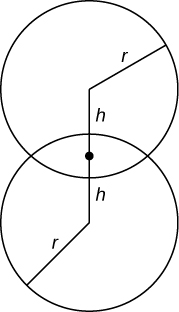$\frac{\pi }{12}{\left(r+h\right)}^{2}\left(6r-h\right)$ units 3

Find the volume of a spherical cap of height $h$ and radius $r$ where $h as seen here.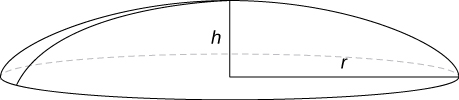Find the volume of a sphere of radius $R$ with a cap of height $h$ removed from the top, as seen here.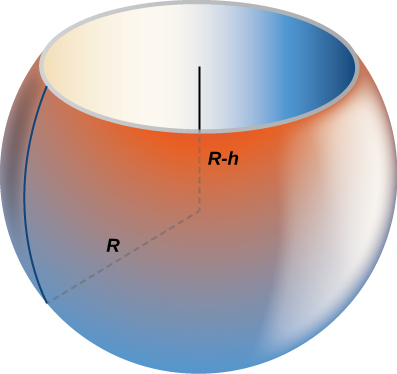$\frac{\pi }{3}\left(h+R\right){\left(h-2R\right)}^{2}$ units 3

how does this work
Can calculus give the answers as same as other methods give in basic classes while solving the numericals?
log tan (x/4+x/2)
Rohan
Rohan
y=(x^2 + 3x).(eipix)
Claudia
Ismael
A Function F(X)=Sinx+cosx is odd or even?
neither
David
Neither
Lovuyiso
f(x)=1/1+x^2 |=[-3,1]
apa itu?
fauzi
determine the area of the region enclosed by x²+y=1,2x-y+4=0
Hi
MP
Hi too
Vic
hello please anyone with calculus PDF should share
Which kind of pdf do you want bro?
Aftab
hi
Abdul
can I get calculus in pdf
Abdul
How to use it to slove fraction
Hello please can someone tell me the meaning of this group all about, yes I know is calculus group but yet nothing is showing up
Shodipo
You have downloaded the aplication Calculus Volume 1, tackling about lessons for (mostly) college freshmen, Calculus 1: Differential, and this group I think aims to let concerns and questions from students who want to clarify something about the subject. Well, this is what I guess so.
Jean
Im not in college but this will still help
nothing
how can we scatch a parabola graph
Ok
Endalkachew
how can I solve differentiation?
with the help of different formulas and Rules. we use formulas according to given condition or according to questions
CALCULUS
For example any questions...
CALCULUS
v=(x,y) وu=(x,y ) ∂u/∂x* ∂x/∂u +∂v/∂x*∂x/∂v=1
log tan (x/4+x/2)
Rohan
what is the procedures in solving number 1?
review of funtion role?
for the function f(x)={x^2-7x+104 x<=7 7x+55 x>7' does limx7 f(x) exist?
find dy÷dx (y^2+2 sec)^2=4(x+1)^2
Integral of e^x/(1+e^2x)tan^-1 (e^x)
why might we use the shell method instead of slicing
fg[[(45)]]²+45⅓x²=100By OpenStaxBy Szilárd JankóBy Jonathan LongBy OpenStaxBy Richley CrapoByBy Stephen VoronBy OpenStaxBy Janet ForresterBy Naveen Tomar